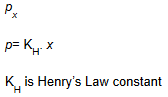# What is Solubility?

The solubility of a substance is the maximum amount that can be dissolved in given amount of solvent at a specific temperature. Factors affecting the solubility:

• Nature of solute
• Nature of solvent
• Temperature
• Pressure

## The solubility of solid in a liquid:

### Nature of solute and solvent:

• According to the nature of solute and solvent, the solubility of solids in a liquid follow the principle “Like dissolves like”.
• If the nature of solute and solvent is same, the intermolecular force of interaction would be same. That helps in solubility of a solute in the solvent.
• Polar solute dissolves in the polar solvent.
For example, NaCl and sugar dissolve in water. Non-polar solute dissolves in a non-polar solvent.
For example, Naphthalene and anthracene dissolve in benzene, not in water.

#### Saturated solution:

If the concentration of solute in solution remains constant at given set of temperature and pressure is called saturated solution. If we add more solute in it, it would precipitate out.

#### Un-Saturated solution:

If the concentration of solute in solution can increase at given set of temperature and pressure is called unsaturated solution. If we add more solute in it, it would get dissolve and increase the concentration of the solution.

### Effect of temperature:

The solubility of the solute in solvent always follows the dynamic equilibrium.

Solute + Solvent Solution

It follows the Le Chateliers principle for the change in temperature at dynamic equilibrium.

• If the solution is formed by giving heat means dissolution is endothermic. By increasing the temperature, the reaction will proceed in forwarding direction and solubility of solute increases.
• If the heat is released in the formation of solute means dissolution is exothermic. By increasing the temperature, the reaction will proceed in backward direction and solubility of solute decreases.

### Effect of Pressure:

Pressure has no significant effect on the solubility of solids in the liquid.

## The solubility of the gas in a Liquid:

### Nature of solute and solvent:

The solubility of a gas in a liquid is also somewhat affected by nature of solute and solvent. Oxygen dissolves only a small extent in water but HCl is highly soluble in water because of polar nature of solute and solvent.

### Effect of pressure:

The solubility of a gas in a liquid is highly affected by temperature and pressure. As the pressure of the gas above the surface of the liquid increases, it increases the solubility of a gas in a liquid. The quantitative relation of this equation is given by Henry’s Law.

### Henry’s Law:

It states that “ At constant temperature, the solubility of a gas in a liquid is directly proportional to the partial pressure of the gas present above the surface of the liquid or solution.”

If we consider mole fraction of a gas in the solution as a measure of its solubility, then it can be said that the “Mole fraction of gas in the solution is proportional to the partial pressure of the gas over the solution”.

Now the Henry’s Law can be stated as “the partial pressure of the gas in the vapour phase (p) is proportional to the mole fraction of the gas (x) in the solution”

#### An expression of Henry’s Law:### Effect of temperature:

Dissolution of gas in a liquid is an exothermic process. As dissolution process involves dynamic equilibrium, it follows Le Chaterlier’s principle. Hence the solubility of a gas in a liquid decreases with increase in temperature.